# Matrices arithmetics help

#### Description

The arithmetic commands in the matrices section of QuickMath allow you to :

• Add one matrix to another
• Subtract one matrix from another
• Multiply two matrices together
• Multiply a matrix by a scalar
• Raise a matrix to a power

To use the matrix arithmetic commands, simply go to the arithmetic page, type in your matrix or matrices, select the command you want to execute and hit the "Calculate" button. Your question will be automatically answered by computer and the reply will be shown in your browser within a few seconds. The answer will be given in two forms : a 'natural' form, which mimics the row and column layout normally used for matrices, and an 'input' form, which is the form required for the entry of matrices into QuickMath. The input form of a solution can be copied and pasted into a matrix input field, so you can use the result from one calculation in another calculation without having to re-type it.

#### Examples

Here are some examples illustrating the types of expressions you can use the factor command on and the results which QuickMath will return.

 Matrix 1 Matrix 2 Operation Result a, b c, d 1, 2 3, 4 MATRIX 1 + MATRIX 2 ```1 + a 2 + b 3 + c 4 + d ``` a, b c, d 1, 2 3, 4 MATRIX 1 - MATRIX 2 ```-1 + a -2 + b -3 + c -4 + d ``` a, b c, d 1, 2 3, 4 MATRIX 1 . MATRIX 2 ```a + 3 b 2 a + 4 b c + 3 d 2 c + 4 d ``` a, b c, d 5 . MATRIX 1 ```5 a 5 b 5 c 5 d ``` a, b c, d MATRIX 1 ^ 2 ``` 2 a + b c a b + b d 2 a c + c d b c + d ```

# MATRIX PRODUCTS

In the last section we multiplied a matrix by a scalar. Multiplication of two matrices is more complicated, but it is important in solving practical problems. To show the reasoning behind matrix multiplication, the example about the vitamin pills from the previous tutorial is continued here.

The matrix below, from the previous section, shows the number of cartons of each type of vitamin received from Dexter and Sullivan, respectively.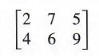To find the total cost of the pills from Dexter, multiply as follows.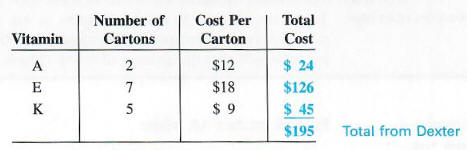The Dexter pills cost a total of \$195.This result is the sum of three products:

2(\$12) + 7(\$18) + 5(\$9) = \$195.

In the same way, using the second row of the matrix and the three costs, the total cost of the Sullivan pills is

4(\$12) + 6(\$18) + 9(\$9) = \$237.

The costs, \$12, \$18, and \$9, can be written as a column matrix.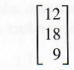The total costs for each supplier, \$195 and \$237, also can be written as a column matrix.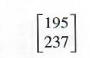The product of the matrices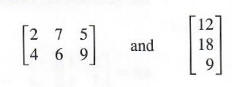can be written as follows.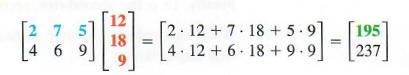Each element of the product was found by multiplying the elements of the rows of the matrix on the left and the corresponding elements of the columns of the matrix on the right, and then finding the sum of these products. Notice that the product of a 2 x 3 matrix and a 3 x 1 matrix is a 2 x 1 matrix.

Generalizing from this example gives the following definition of matrix multiplication.

The product AB of an m x n matrix A and an n x k matrix B is found as follows. To get the ith row, jth column element of AB, multiply each element in the ith row of A by the corresponding element in the jth column of B. The sum of these products will give the element of row i, column j of AB.

Example 1

Find the product AB, where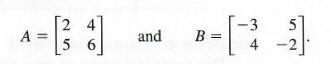Step 1 Multiply the elements of the first row of A and the corresponding elements of the first column of B, and add these products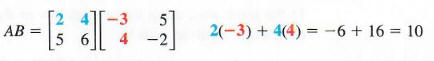The first-row, first-column entry of the product matrix AB is 10.

Step 2 Multiply the elements of the first row of A and the second column of B and then add the products to get the first-row, second-column entry of the product matrix.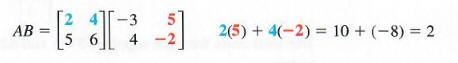Step 3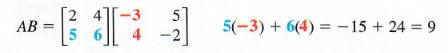The second-row, first-column entry of the product matrix is 9.

Step 4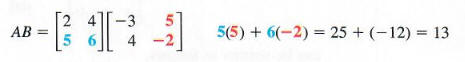Finally, 13 is the second-row, second-column entry.

Step 5 Write the product. The four entries in the product matrix come from the four steps above.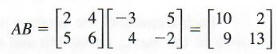RESTRICTION ON MATRIX MULTIPLICATION

The product AB of two matrices A and B can be found only if the number of columns of A is the same as the number of rows of B. The final product will have as many rows as A and as many columns as B.

DECIDING WHETHER TWO MATRICES CAN BE MULTIPLIED

Suppose matrix A is 2 X 2, while matrix B is 2 X 4. Can the product AB be calculated? What is the size of the product?

The following diagram helps answer these questions.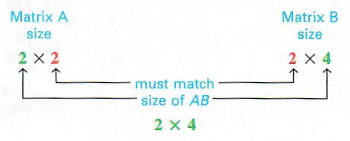The product AB can be calculated because A has two columns and B has two rows. The size of the product is 2 X 4. (However, the product BA could not be found.)

MULTIPLYING MATRICES IN DIFFERENT ORDERS

Find AB and BA if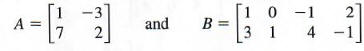Use the definition of matrix multiplication to find AB.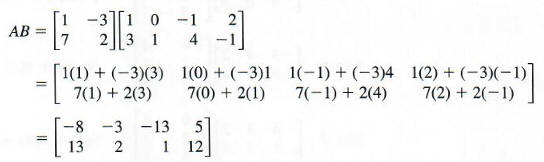Since B is a 2 x 4 matrix, and A is a 2 X 2 matrix, the product BA cannot be found.

MULTIPLYING SQUARE MATRICES IN DIFFERENT ORDERS

Find MN and NM, given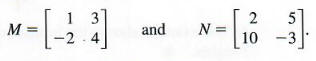By the definition of matrix multiplication,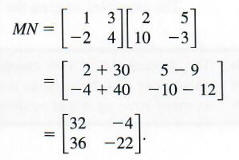Similarly,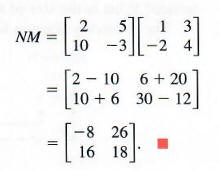ln the above example , although both MN and NM could be found, they were not equal, showing that multiplication of matrices is not commutative. This fact distinguishes matrix arithmetic from the arithmetic of real numbers.